# Green equivalence relations

(diff) ← Older revision | Latest revision (diff) | Newer revision → (diff)
Binary relations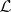,,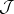,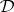,defined as follows: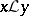means thatandgenerate identical left principal ideals (cf. Principal ideal);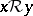and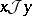have a similar meaning after "left" has been replaced by "right" and "two-sided" , respectively;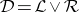(union in the lattice of equivalence relations);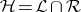. The relationsandare commutative in the sense of multiplication of binary relations, so thatcoincides with their product. The relationis a right congruence, i.e. is stable from the right: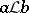implies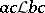for all; the relation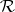is a left congruence (stable from the left). An-class and an-class intersect if and only if they are contained in the same-class. All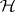-classes in the same-class are equipotent. If a-classcontains a regular element, then all elements inare regular andcontains with some given element all elements inverse to it; such a-class is said to be regular. In a regular-class each-class and each-class contains an idempotent. Letbe an arbitrary-class; then eitheris a group (which is the case if and only ifis a maximal subgroup of the given semi-group), or else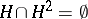. All group-classes of the same-class are isomorphic groups. In the general case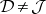, but if, for example, some power of each element of the semi-groupbelongs to a subgroup (in particular, ifis a periodic semi-group), then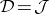. The inclusion of principal left ideals defines in a natural manner a partial order relation on the set of-classes; similar considerations are valid for-classes and-classes. These relations were introduced by J. Green .